Next: Singular Value Decomposition as Up: Singular System Analysis Previous: Singular System Analysis   Contents

### Choice of a Proper Embedding Dimension for a Noise-Free Time Series of Finite Length

As a first step we choose some. We want to embed
in
. In generalwill be unknown so that it is not obvious how to meet Takens' condition
. But if we chooselarge enough to ensure thatthen we can embedin, according to Takens' theorem 2, because it is a well known fact that every Euclidean space
can again be embedded in any higher-dimensional Euclidean spacewithout any problems. So we start by making an ansatz forand our concern for the next few paragraphs will be to find a more appropriate
such that
is a space containing Takens' embedding space. Thus
will be a better embedding dimension than thewe started with, since it gives a lower-dimensional embedding space. We want to use the method of delays to construct vectors infrom the
of the time series. Doing this one realizes that there is even one more quantity which is not yet specified: One could, for example, take the series of-vectors(27)

which we are obviously allowed to use in accordance with Takens' statements. So we also have to choose the lag time''
, where
. We will see in section 3.4.2 that we do not have to spend much effort on choosing
: when we use the singular systems technique the influence of the lag time becomes insignificant, hence we will choose it from now on to equal
(i.e.). Consider a sequence of
vectors,
(i.e. we take a time series containing
data points)12. There seems to be no analytical way to compute the proper (i.e. minimal) embedding dimension from the time series. However, it is possible to determine a reasonable estimate for it: For some giventhe-vectors
usually do not explore the whole space. Rather than that they are restricted to some subspace
of; T contains the embedded manifoldwhich contains the picture of the attractor:
(28)

When we assume that thereally visit the whole attractor in the embedding space (more or less) uniformly and we bear in mind thatusually is much larger thanthen
is a sensible upper bound for the minimal embedding dimension. In order to determinewe compute the maximum number of linearly independent vectors that can be constructed as linear combinations of the. To do this, we define the
-trajectory matrix
: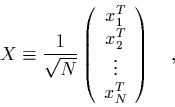(29)

which is built out of all vectors we want to use to reconstruct the attractor. Notice that when operating withon some-vector we get an-vector:(30)

Since we are interested in linearly independent-vectors, we choose a set of vectors
such that the-vectors(31)

are orthonormal. We introduce some real constants
into this equation, in order to normalize the
:
(32)

The important point about this equation is that, after transposing, it can be rewritten as
(33)

i.e. as a linear combination of the reconstructed trajectory vectors. This tells us, when we keep in mind the definition of, that we can getlinearly independent vectors
, using eq. (34); so we havevectors
andnumbers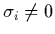, too. The
are elements of an orthonormal basis
of. Thus we are left to determineas the number of those
which are non-zero. Define the structure matrix; then it follows from eq. (34) that theare the eigenvalues of this matrix:
(34)

We could determineas the number of the non-zero eigenvalues of. Butis a huge
-matrix, and singular, and its diagonalization is in practice impossible. Instead, we notice that the covariance matrix
has the same non-zero eigenvalues as, and
is much easier to diagonalize, because it is only an
-matrix. So all one has to do in order to calculatewhich, cum grano salis, estimates the minimal embedding dimension
, is to determine the number of the non-zero eigenvalues of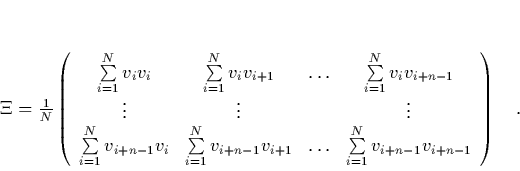(35)

Now we know that the trajectory is confined to an-dimensional subspace of, and we can useas the embedding space. However, this treatment only makes sense in the case that we have noise- free data.

#### Footnotes

... points)12
We will generally assume
, which is obviously true in most cases.Next: Singular Value Decomposition as Up: Singular System Analysis Previous: Singular System Analysis   Contents
Martin_Engel 2000-05-25